Lecture 7
Example of Between Groups t-test

As an example, let us consider a hypothetical research study that examines dispositional characteristics of children born first or second in a family. Twenty children are surveyed on their level of introversion to see if children born first are more likely to be introverted than children born second (perhaps because they don't have a sibling to play with in the first couple of years). The scores on the introversion test have possible values of 0 to 100, with higher scores indicating more introversion. Here are the data for our sample of twenty:

 Child ID Born first or second (1=first, 2=second) Introversion score 1 1 65 2 1 48 3 1 63 4 1 52 5 1 61 6 1 53 7 1 63 8 1 70 9 1 65 10 1 66 11 2 61 12 2 42 13 2 66 14 2 52 15 2 47 16 2 58 17 2 65 18 2 62 19 2 64 20 2 69

Conducting the Significance Test
I'm going to cheat a little and assume that the variances and means for each have already been computed: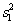= 50.93 and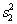= 78.27 and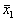= 60.6 and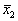= 58.6.

We start by figuring out the pooled variance estimate where the variances for the two groups are put together. For that we use this formula,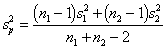in whichare the variances for the two groups, and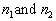are the sample sizes for each group. Plugging these numbers in we get: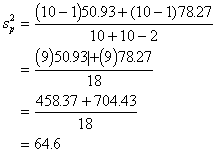Now, to the standard error, whose formula looks like this: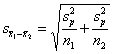And plugging in the values we already have,Since we now have the standard error and we were given the means for the two groups before, we can get the t-value.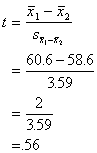To see if the difference between the means is extreme enough to be significant at a = .05, we look up in the t-table in the back of the text (Table E) under d.f. = n1 + n2 - 2 = 10 + 10 - 2 = 18, using the column t.975 because we are doing a two-tailed test with a = .05. The corresponding number in the body of the table is 2.1009. Our calculated t-value, .56, does not excede this number from the table, so it is not extreme enough. We conclude that the difference between the two groups is nonsignificant at p < .05. In other words, we decide to retain the null hypothesis that says that, in the population, first and second children are equally introverted.

Confidence Intervals
We could have also done this test by calculating the 95% confidence interval and checking to see if we would predict that the group difference in the population would be greater than zero. To do this, we use the previously obtained values to compute the LCL and UCL (upper and lower confidence levels). The formula for the confidence intervals looks like this: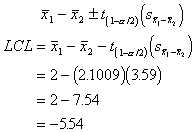The UCL is pretty easy once we've done this: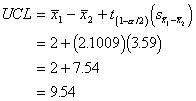The confidence limits are -5.54 and 9.54, and because they contain the number 0, that means that the difference between the two group means in the population could be 0. So, we can't be sure that there will be a difference in the population. That leads us to the same conclusion, that in the sample the group means are not significantly different from one another.